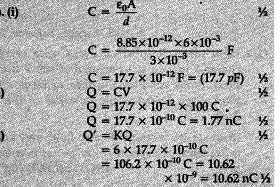# In a parallel plate capacitor with air between the plates, each plate has an area of and the separation between the plates is 3mm

In a parallel plate capacitor with air between the plates, each plate has an area of and the separation between the plates is 3mm.
(i) Calculate the capacitance of the capacitor.
(ii) If this capacitor is connected to 100 V supply, what would be the charge on each plate ?
(iii) How would charge on the plates be affected, if a
3 mm thick mica sheet of K = 6 is inserted between the plates while the voltage supply remains connected ?